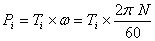### Engine formulas

Cylinder Swept Volume (Vc):where:
Vc= cylinder swept volume [cm3 (cc) or L]
Ac = cylinder area [cm2 or cm2/100]
dc = cylinder diameter [cm or cm/10]
L = stroke length (the distance between the TDC and BDC) [cm or cm/10]

* Increase the diameter or the stroke length will increase the cylinder volume, the ratio between the cylinder diameter/cylinder stroke called “bore/stroke” ratio.
- “bore/stroke” >1 is called over square engine, and is used in automotive engines
- “bore/stroke” =1 is called square engine
- “bore/stoke” <1 is called= under square engine, and is used in tractor engine

Engine Swept Volume (Ve):where:
Ve = engine swept volume [cm3 (cc) or L]
n = number of cylinders
Vc = cylinder swept volume [cm3 (cc) or L]
Ac = cylinder area [cm2 or cm2/100]
dc =  cylinder diameter [cm or cm/10]

* The units of cylinder swept volume is measured in (cm3, cubic centimeter (cc), or liter)
- Ve  for small engines, 4 cylinder engines is (750 cc:1300 cc)
- Ve for big engine, 8 cylinder engines is (1600 cc:2500 cc)

Compression Ratio (r):where:
r = compression ratio
Vs = cylinder swept volume (combustion chamber volume) [cc, L, or m3]
Vc = cylinder volume     [cc, L, or m3]

* Increase the compression ratio increase engine power
- r (gasoline engine) = 7:12, the upper limit is engine pre ignition
- r (diesel engine) = 10:18, the upper limit is the stresses on engine parts

Engine Volumetric Efficiency (hv):where:
hV  = volumetric efficiency
Vair = volume of air taken into cylinder [cc, L, or m3]
Vc = cylinder swept volume [cc, L, or m3]

* Increase the engine volumetric efficiency increase engine power
- Engine of normal aspiration has a volumetric efficiency of 80% to 90%
- Engine volumetric efficiency can be increased by using:
(turbo and supper charger can increase the volumetric efficiency by 50%)

Engine Indicated Torque (Ti):where:
Ti = engine indicated torque [Nm]
imep = indicated mean effective pressure [N/m2]
Ac = cylinder area [m2]
L = stroke length [m]
z = 1 (for 2 stroke engines), 2 (for 4 stroke engines)
n = number of cylinders
θ = crank shaft angle [1/s]

Engine Indicated Power (Pi):,where:
imep = is the indicated mean effective pressure [N/m2]
Ac = cylinder area [m2]
L = stroke length [m]
n = number of cylinders
N = engine speed [rpm]
z = 1 (for 2 stroke engines), 2 (for 4 stroke engines)
Vc = cylinder swept volume [m3]
Ve = engine swept volume [m3]
Ti = engine indicated torque [Nm]
ω = engine angular speed [1/s]

Engine Mechanical Efficiency (hm):where:
hm = mechanical efficiency
Pb = engine brake power [kW]
Pi = engine indicated power [kW]
Pf = engine friction power [kW]

Engine Specific Fuel Consumption (SFC):where:
SFC = specific fuel consumption [(kg/h)/kW, kg/(3600 s x kW), kg/(3600 kJ)]
FC = fuel consumption [kg/h]
Pb = brake power [kW]

Engine Thermal Efficiency (hth):where:
hth = thermal efficiency
Pb = brake power [kW]
FC = fuel consumption [kg/h = (fuel consumption in L/h) x (ρ in kg/L)]
CV = calorific value of kilogram fuel [kJ/kg]
ρ = relative density of fuel [kg/L]

Disclaimer: